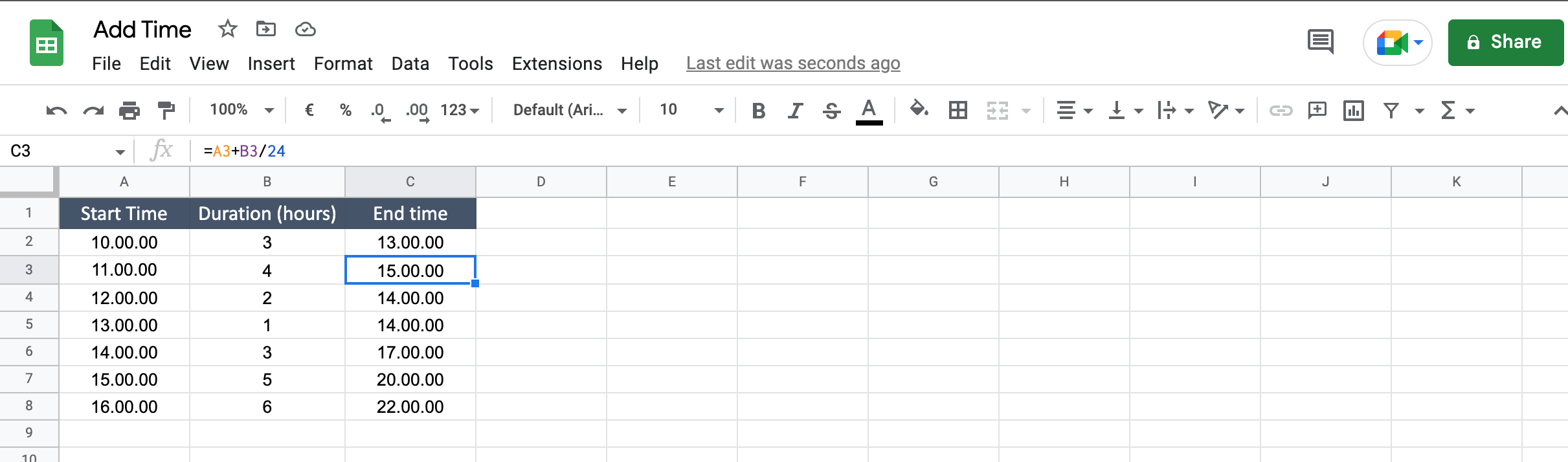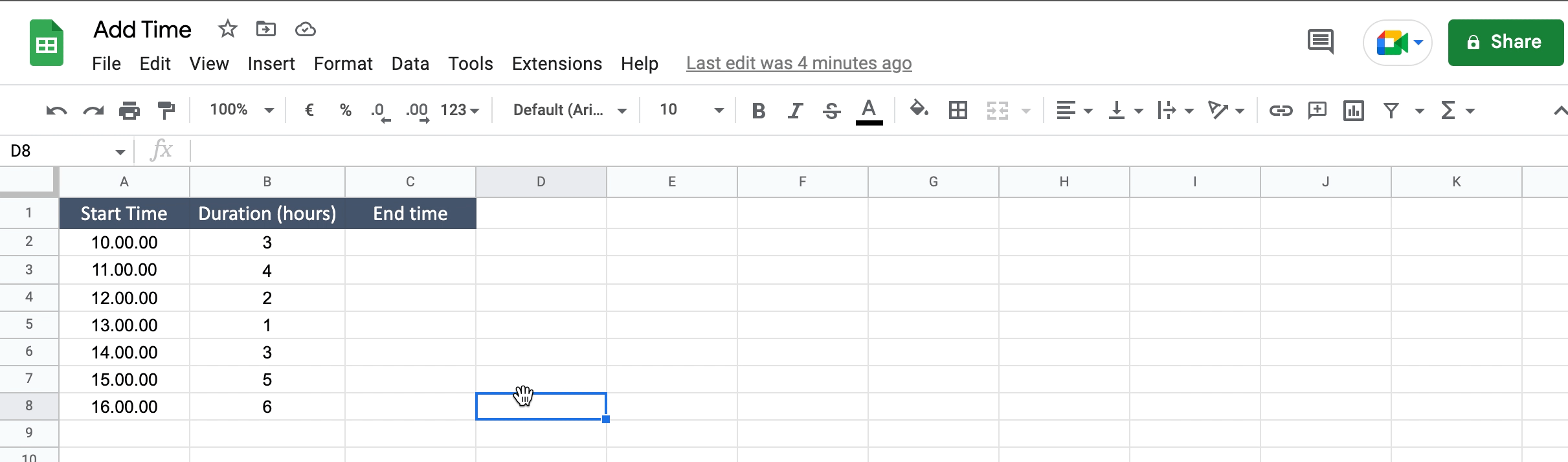Sometimes you have to deal with time data, like train schedule, working hours, travel plan, etc. And maybe you have to add time from a starting point to calculate an arrival or and ending time. To do that you can follow the steps below.

### Step 1 – Select the cell where you will insert the sum– Click on the cell where you will write the formula.

### Step 2 – Write the formula– Type “=” to start the formula;
– Select the cell where you have the starting time;